# 数据结构与算法（图）

7 篇文章 2 订阅

#### 首语

• 二叉树的删除（扩展）
/**
* 删除结点
* @param key
*/
public void deleteNode(int key) throws Exception {
TreeNode node=searchNode(key);
if (node==null){
throw new Exception("该结点不存在！");
}else {
//删除该结点
delete(node);
}
}

private void delete(TreeNode node) throws Exception {
if (node==null){
throw new Exception("该结点不存在！！！");
}else {
TreeNode parent=node.parent;
//叶子结点
if (node.leftChild==null&&node.rightChild==null){
if (parent.leftChild==node){
parent.leftChild=null;
}else {
parent.rightChild=null;
}
}else if (node.leftChild!=null&&node.rightChild==null){
if (parent.leftChild==node){
parent.leftChild=node.leftChild;
}else {
parent.rightChild=node.leftChild;
}
}else if (node.leftChild==null&&node.rightChild!=null){
if (parent.rightChild==node){
parent.leftChild=node.rightChild;
}else {
parent.rightChild=node.rightChild;
}
}else {
//该结点的后继结点
TreeNode next=getNextNode(node);
delete(next);
node.data=next.data;
}
}
}

private TreeNode getNextNode(TreeNode node) {
if (node==null){
return null;
}else {
if (node.rightChild!=null){
//找某结点最小关键字结点
return getMinTreeNode(node.rightChild);
}else {
TreeNode parent=node.parent;
while (parent!=null&&node==parent.rightChild){
node=parent;
parent=parent.parent;
}
return parent;
}
}
}

private TreeNode getMinTreeNode(TreeNode node) {
if (node==null){
return null;
}else {
while (node.leftChild!=null){
node=node.leftChild;
}
}
return node;
}


#### 图

• 图（Graph）是由顶点的有穷非空集合和顶点之间边的集合组成，通常表示为：G（V,E）其中，G表示一个图，V是图G中顶点的集合，E是图G中边的集合。

#### 图的特性

• 线性表中我们把数据元素叫元素，树中将数据元素叫结点，在图中数据元素，我们称之为顶点（Vertex）。
• 线性表中可以没有数据元素，称为空表。树中可以没有结点，叫做空树。
• 线性表中，相邻的数据元素之间具有线性关系，树结构中，相邻两层的结点具有层次关系，而图中，任意两个顶点之间都可能有关系，顶点之间的逻辑关系用边表示，边集可以是空的。

#### 无向图

• 无向边：若顶点vi到vj之间的边没有方向，则称这条边为无向边（Edge），用无序偶对（vi，vj）来表示。如果图中任意两个顶点之间的边都是无向边，则称该图为无向图（Undirected graphs）。
• 在无向图中，如果任意两个顶点之间都存在边，则称该图为无向完全图。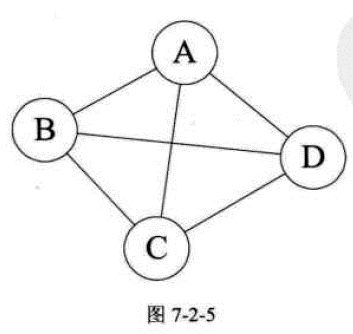#### 有向图

• 有向边：若从顶点vi到vj的边有方向，则称这条边为有向边，也称为弧（Arc）。
• 在有向图中，如果任意两个顶点之间都存在方向互为相反的两条弧，则称该图为完全有向图。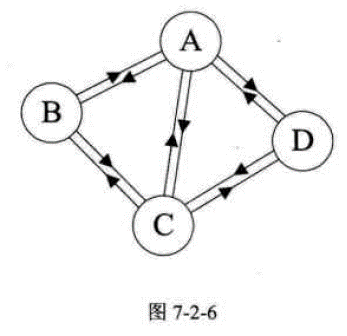#### 图的权（Weight）

• 有些图的边或弧具有与它相关的数字，这种与图的边或弧相关的数叫做权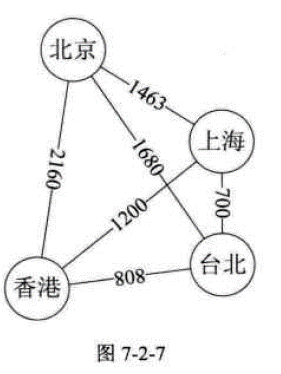#### 连通图

• 在无向图G中，如果从顶点v到顶点v’有路径，则称v和v’是连通的。如果对于图中任意两个顶点vi、vj∈E，vi和vj都是连通的，则称G是连通图（Connected Graph）。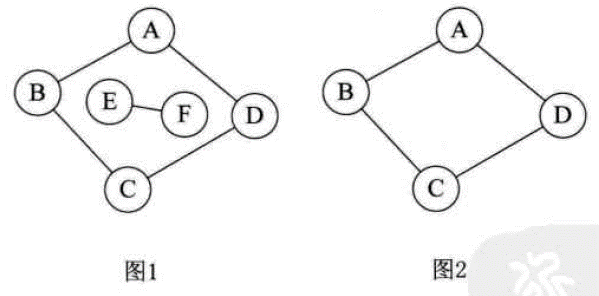#### 度

• 无向图顶点的边数叫度，有向图顶点的边数叫出度和入度。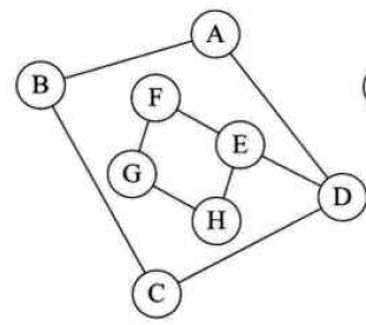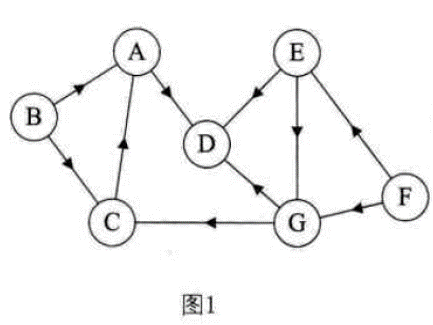#### 图的存储结构

• 也正由于图的结构比较复杂，任意两个顶点之间都可能存在关系，因此无法以数据元素在内存中的物理位置来表示元素之间的关系，也就是说，图不可能用简单的顺序存储结构来表示。下面表示同一个图。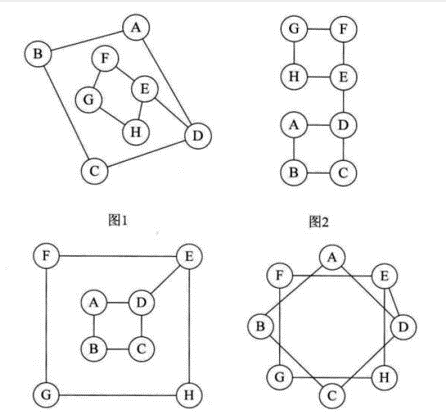#### 邻接矩阵

• 考虑到图是由顶点和边或弧两部分组成。合在一起比较困难，那就很自然的考虑到分两个结构来分别存储。顶点不分大小、主次，所以用一个一维数组来存储是很不错的选择。而 边或弧由于是顶点与顶点之间的关系，一维搞不定，那就考虑用一个二维数组来存储。于是我们的邻接矩阵的方案就诞生了。
• 无向图的边数组是一个对称矩阵。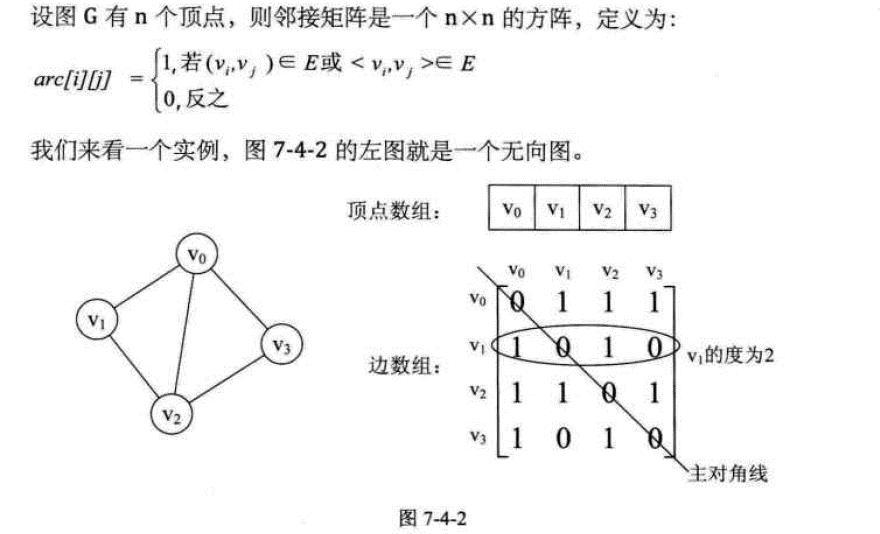• 对于有向图，水平方向出度，水平方向入度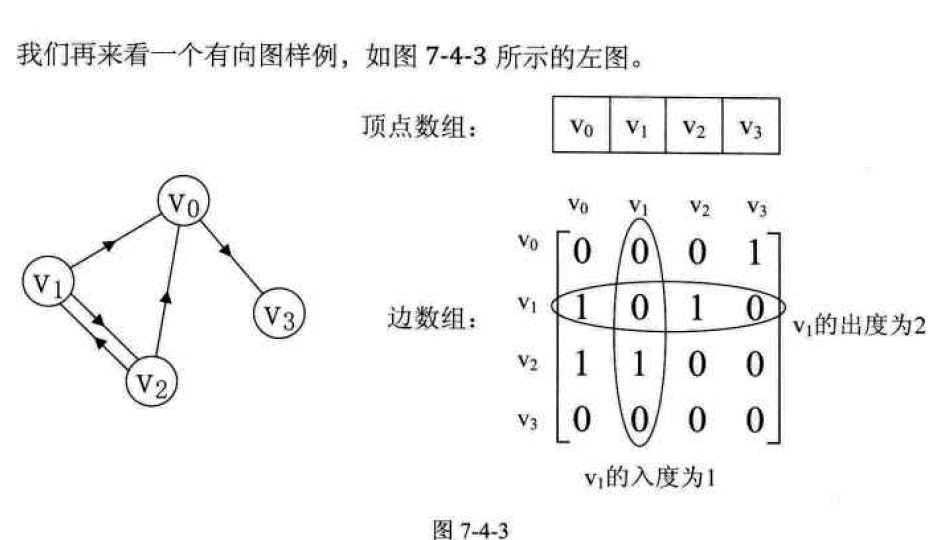#### 带权邻接矩阵#### 有向图出度计算

	private int vertexSize;//顶点数量
private int [] vertexs;//顶点数组
private int [][] matrix;
private static final int MAX_WEIGHT=1000;

public Graph(int vertexSize) {
this.vertexSize=vertexSize;
matrix=new int[vertexSize][vertexSize];
vertexs=new int[vertexSize];
for(int i=0;i<vertexSize;i++){
vertexs[i]=i;
}
}
Graph graph = new Graph(5);
int[] a1 = {0, MAX_WEIGHT, MAX_WEIGHT, MAX_WEIGHT, 6};
int[] a2 = {9, 0, 3, MAX_WEIGHT, MAX_WEIGHT};
int[] a3 = {2, MAX_WEIGHT, 0, 5, MAX_WEIGHT};
int[] a4 = {MAX_WEIGHT, MAX_WEIGHT, MAX_WEIGHT, 0, 1};
int[] a5 = {MAX_WEIGHT, MAX_WEIGHT, MAX_WEIGHT, MAX_WEIGHT, 0};
graph.matrix=a1;
graph.matrix=a2;
graph.matrix=a3;
graph.matrix=a4;
graph.matrix=a5;

/**
* 图出度计算
* @param index
*/
public int getOutDegree(int index){
int degree=0;
for (int j=0;j<matrix[index].length;j++){
int weight=matrix[index][j];
if (weight!=0&&weight!=MAX_WEIGHT){
degree++;
}
}
return degree;
}


#### 有向图入度计算

/**
* 图入度计算
* @param index
*/
public int getInDegree(int index){
int degree=0;
for (int i=0;i<matrix[index].length;i++){
int weight=matrix[i][index];
if (weight!=0&&weight!=MAX_WEIGHT){
degree++;
}
}
return degree;
}


#### 两个顶点之间的权值计算

/**
* 获得两个顶点之间的权值
* 0:本身；-1无边
* @param v1
* @param v2
* @return
*/
public int getWeight(int v1,int v2){
return matrix[v1][v2]==0?0:(matrix[v1][v2]==MAX_WEIGHT?-1:matrix[v1][v2]);
}


#### 浪费的邻接矩阵

• 讲到了一种孩子表示法，将结点存入数组，并对结点的孩子进行链式存储，不管有多少孩子，也不会存在空间浪费问题，这个思路同样适用于图的存储，我们把这种数组与链表相结合的存储方法叫做邻接表。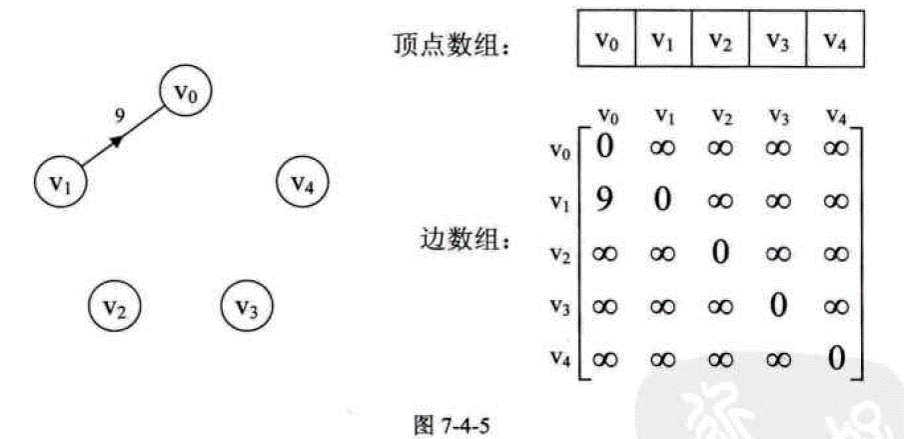#### 无向图的邻接表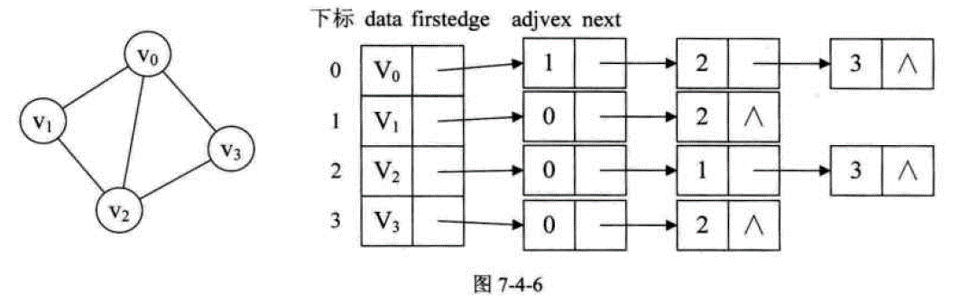#### 有向图的邻接表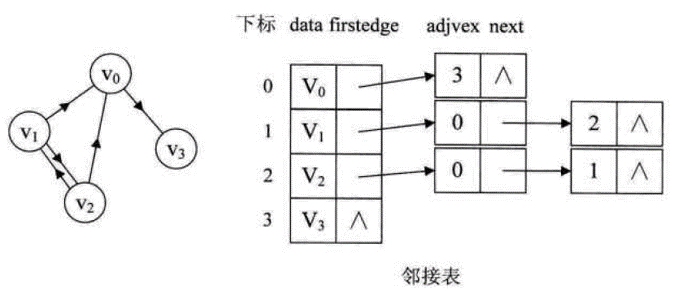#### 逆邻接表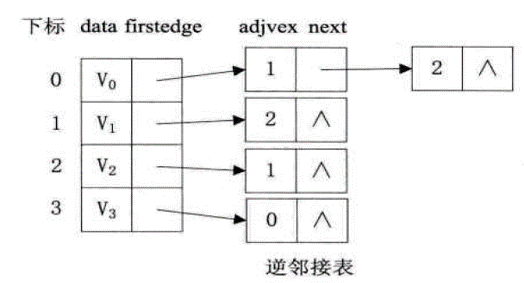#### 带权值邻接表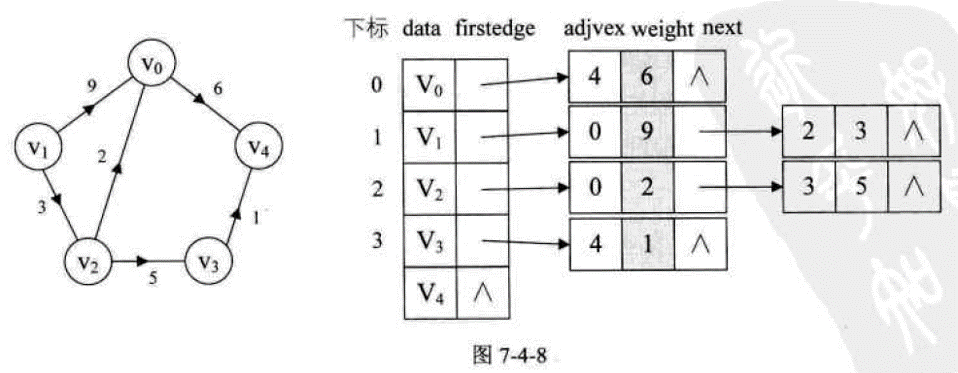05-20
08-04316604-25357
04-11429
01-042624
06-191293
07-0713
08-101225
02-193504
03-301226
03-25908
02-08218
08-181万+
10-02645
04-12216
12-05点击重新获取扫码支付余额充值